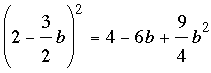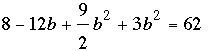SEARCH HOMEMath Central Quandaries & QueriesQuestion from Sabbie, a student: Hi, I need help solving this equation for my physics homework,so I can solve the quadratic equations for x and y. I've derived the following equations from the information provided in the question. 2a+3b = 4 -- 1 2a^2 + 3b^2 = 62 -- 2 a=2-(3/2)b is substituted into equation 2. so, i get 2 (2-(3/2)b)^2 + 3b^2 = 62 this is where the problem begins. i cannot work through this to get to the correct quadratic equation 5b^2-8b-36=0 I can't seem to be able to figure out where I keep going wrong. I would really appreciate it if you could give me a step-by-step breakdown of the workout. thanks!Sabbie,

Expanding (2-(3/2)b)^2 I getand thus the equation becomesI then multiplied both sides by 2 and collected terms to get

15b2 - 24b - 108.

Finally dividing both sides by 3 gives the desired equation.

PennyMath Central is supported by the University of Regina and The Pacific Institute for the Mathematical Sciences.